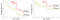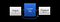# Intuition For: ResNet — Deep Residual Learning for Image Recognition

“Deep Residual Learning for Image Recognition” was published on Dec 10, 2015 and as of today, it is one of the most cited papers in machine learning. Surprisingly, it is also one of the easiest concepts to understand as it does not require any knowledge beyond simple neural networks. In this article, we will intuitively explain the core concept of ResNet without all the unnecessary terms and symbols.

# Problem

Theoretically, the more layers you add to a neural network, the performance could either go up or stay the same, it should never go down. Therefore, to make a neural network better, just add more layers!

Here’s an example:

Suppose Neural Net #1 has achieved 100% accuracy and its loss function is at the global minimum; in other words, the neural network is in its best possible state. Now as you add more hidden layers as seen in Neural Net #2, theoretically the new layers should learn the identity function(mapping the input directly to the output, e.g. g(x)= x) to preserve the current best possible state of the network — these 2 networks are essentially equivalent.

However, experimentally, learning the identity function is extremely difficult as the scope of all possible combination of weights and biases is enormous, thus the chance of learning the identity function is minuscule.Training error (left) and test error (right) on CIFAR-10 with 20-layer and 56-layer “plain” networks. The deeper network has higher training error, and thus test error.

As seen above, adding more layers to a neural network can actually do the opposite: more layers = lower accuracy(diminishing returns).

So although theoretically, as you add extra layers, those layers should just learn the identity function; however, experimentally, learning the identity function is way too difficult in a finite amount of time and data — it converges way too slow. This problem is known as degradation, and it’s a major roadblock in building deeper networks.

Then the question must be asked: is there any way to make learning the identity function easier, so you can add more layers without diminishing returns?

# Solution

Yes, there is a way! And it’s done by adding the input of hidden layer(s) to their output.

How does that work? Good question. The goal here is to learn the identity function, which for simplicity sake, let’s say the hidden layer only consists of a single weight. Therefore, with a regular neural network the goal is to get that weight to be 1(because 1 x input = input, duh!). Now as seen beforehand, that doesn’t work well experimentally…*f(x) does not have to be one hidden layer, could be any arbitrary number of hidden layers

By adding the input to the output as seen above, instead of trying to learn the identity function, you want to learn the “zero” function(0 x input + input = input, duh). Because the identity function is already there — the input is directly passed to the output! The “zero” function, f(x) = 0, is much easier to learn therefore it does not suffer from the same degradation problem.

Let’s encapsulate the entire function above: f(x) + x, as g(x). Now, what happens if you don’t want to learn the identity function, what if you want to use this architecture for a general neural network?

The neural network can still train and learn normally! For its hidden layers, it learns g(x) - x. If this isn’t crystal clear, here’s some algebra: f(x) + x = g(x), f(x) = g(x) - x.

Another way to think about it is: if you initialize a regular neural network’s weights and biases to be 0 at the start, every layer starts with the “zero” function. In contrast, this neural network essentially starts with the identity function since the input is passed directly to the output — it learns what’s leftover(residuals) after the identity function is added. Hence the name, residual network, ResNet!

And indeed, ResNet solves the degradation problem, which allows it to take advantage of adding hundreds of new layers without diminishing returns.

# Conclusion

To summarize, regular neural networks suffer from the degradation problem because of the infeasibility to learn the identity function. ResNet solves degradation by adding the input of a layer to its output, therefore easing the process of learning the identity function since it’s “already there”.

The Paper: Deep Residual Learning for Image Recognition
Authors:
Kaiming He, Xiangyu Zhang, Shaoqing Ren, Jian Sun

👋 Hey! I’m a 16-year-old entrepreneur, & Machine Learning/Python/C++/Web developer located in Toronto.

If you have any suggestions, ideas for projects, or just want to connect, feel free to message me on LinkedIn(http://linkedin.com/in/realmichaelye) or email realmichaelye@gmail.com!

Written by